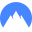# HTML5 - Canvas: Draw pictures and manipulate pixelsOctaFX Broker No Swap, Top 1 Malaysia, IndonesiaIQ Option Top 1 binary option, Give \$10,000 demo accountVultr VPS Start \$2.50/month. Give \$100 todayNord VPN Get 70% off

Want to create special visual effects when using canvas, you can not just use individual attributes and methods available on the object context. Therefore, this article will show you how to draw and manipulate images from the object pixel ImageData.

To draw a picture off the canvas, create an object image and implement methods context.drawImage () in the event of image load. So to ensure that images are only loaded drawing after completion. Additionally, you should put the load event before assigning image links. If no image can be loaded before you attach the load event for it.

The method drawImage () has three overloaded follows:

- Draw the image at a position destX, destY:

`context.drawImage (image, destX, destY);`

- Draw image at destX location, and size destY destWidth, destHeight:

`context.drawImage (image, destX, destY, destWidth, destHeight);`

- Cut the image at the position [sourceX, sourceY, sourceWidth, sourceHeight] and draw at [destX, destY, destWidth, destHeight]:

```context.drawImage (image, sourceX, sourceY, sourceWidth,

sourceHeight, destX, destY, destWidth, destHeight);```

For example:

 `window.onload = ``function ``() {` `    ``var ` `canvas = document.getElementById ( ``"mycanvas" ``);``    ``var ` `context = canvas.getContext ( ``"2d" ``);` `    ``var ` `img = ``new ` `Image ();` `    ``img.onload = ``function ``() {``        ``context.drawImage (img, 10, 10,50,50);``    ``};``    ``img.src = ``"foo.png" ``;``};`

# Manipulating pixels

An image consists of an array of pixels with values ​​red, green, blue and alpha (RGBA). Which is the value determined alpha opacity (opacity) of the image. The larger the value of alpha color becomes clearer and the colors will become transparent if alpha is 0.

In the Canvas 2D API, image data is stored in an object with 3 properties ImageData the width, height and data. Which data is a one-dimensional array containing the pixel. Each pixel contains four elements corresponding to R, G, B, A.

Thus with an image size of 10 × 20 pixels and will have 200 200 * 4 = 400 elements in the array ImageData.data.You can refer to the information on this API tại: https://www.whatwg.org/specs/web-apps/current-work/multipage/the-canvas-element.html#pixel-manipulation :

imagedata = context.createImageData (sw, sh)

Returns an object with size ImageData sw x sh. All of this object pixel is black throughout.

imagedata = context.createImageData (imagedata)

Returns object ImageData  with size equal to the object in the parameter. All pixels are black throughout.

imagedata = context.getImageData (sx, sy, sw, sh)

Returns an object containing data ImageData rectangular image area (defined by the parameters) of the canvas.

NotSupportedError throw exception if there is any parameter that is not a valid number. Throw width or height IndexSizeError exception if zero.

imagedata.width

imagedata.height

Returns the actual size of the object ImageData, in pixels.

imagedata.data

Returns dimensional array data RGBA format, each value is between 0 and 255.

context. putImageData (imagedata, dx, dy [, dirtyX, dirtyY, dirtyWidth, dirtyHeight])

Drawing data from objects on the canvas at the location ImageData dx, dy. If the rectangle (from the parameters dirtyX, dirtyY, dirtWidth, dirtyHeight) is determined, the data portion of this ImageData new rectangular area is drawn on canvas.These properties determine the effect of context draw will be ignored when this method is called. The pixels from the canvas will be replaced entirely by ImageData without the combination of colors, effects, ... with the image data available on the canvas.

One common example is the most simple and the color of the image reversed. This is done by taking the maximum color value (255) minus the value of each RGB color channel of each pixel current. Alpha value to the maximum value for the clearest image.

 `< ``html ``>``< ``head ``>``< ``script ``>``    ``window.onload = function () {` `        ``var img = new Image ();``        ``img.onload = function () {``            ``invertColor (this);``        ``};``        ``img.src = "panda.jpg";``    ``};``    ``invertColor function (img) {``        ``var canvas = document.getElementById ("mycanvas");``        ``var context = canvas.getContext ("2d");` `        ``// Draw the image at the top-left corner``        ``context.drawImage (img, 0,0);``        ``// Draw original image Beside the previous right image``        ``context.drawImage (img, img.width, 0);` `        ``// Get the object from the left image ImageData``        ``var imageData = context.getImageData (0, 0, img.width, img.height);``        ``var data = imageData.data;` `        ``for (var i = 0; i < ``data.length ``; i + = 4) {` `            ``data [i] = 255 - data [i]; // Red``            ``data [i + 1] = 255 - data [i + 1]; // Green``            ``data [i + 2] = 255 - data [i + 2]; // Blue``            ``data [i + 3] = 255; // Alpha``        ``}` `        ``context.putImageData (imageData, 0.0);` `    ``}``````< ``body ``>``   ``< ``canvas ` `id ``= ``"mycanvas" ` `width ``= ``"600" ` `height ``= ``"250" ``> `````

Result:You can add parameters to create a "dirty rectangle" in method putImageData () if you want to draw up a rectangular area ImageData determination of canvas.

For example, I choose the same rectangular area on ImageData and drew up two different rectangular region on the canvas to get the following result:

```context.putImageData (imageData, 0,0,0,0, img.width / 2, img.height / 2);
context.putImageData (imageData, img.width, 0,0,0, img.width / 2, img.height / 2);```OctaFX Broker No Swap, Top 1 Malaysia, IndonesiaIQ Option Top 1 binary option, Give \$10,000 demo accountVultr VPS Start \$2.50/month. Give \$100 todayNord VPN Get 70% off